# Embedded Figures: Basic Concept, Types of Embedded Figure Questions, Tips & Tricks to Solve Embedded Figure QuestionsPosted on : 25-12-2018 Posted by : Admin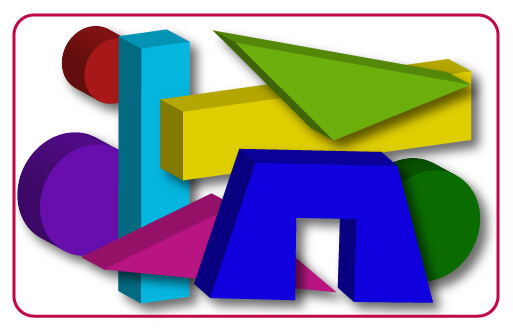## Embedded figure meaning

Embedded figures are the figures hidden inside another figure. In other words, a figure (x) is said to be embedded inside figure (y), if figure (y) contains figure (x) in it.

Embedded figure questions consist of unique figure which is hidden or embedded in one of the four option figures. In such questions, all the options look same and confusing. So candidates need to be careful while attempting such questions. Anyhow with practice we can master these embedded figures. We must basically understand the structure of the given question figure and then proceed to find the correct answer figure.

## Types of embedded figure questions

There are many types of embedded figure questions. A few of them are explained below,

### Type 1: Complex answer figure

A question figure (x) is given followed by four complex option figures in such a way that the question figure (x) is embedded in only one of the given four options. The candidate must identify the correct figure in which figure (x) is hidden.

Example 1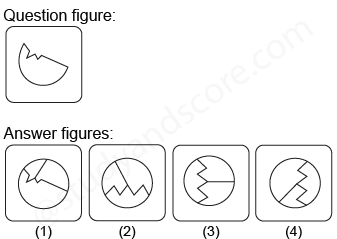Solution: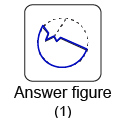Example 2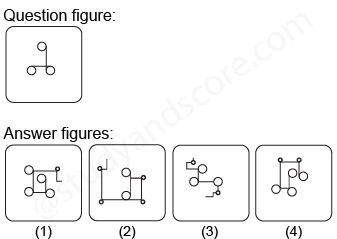Solution: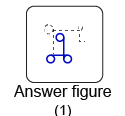### Type 2: Complex question figure

A complex question figure (x) is given followed by four option figures in such a way that only one of the option figures is embedded inside the given question figure. The candidate must identify the correct option figure which is hidden inside the question figure (x).

Example 1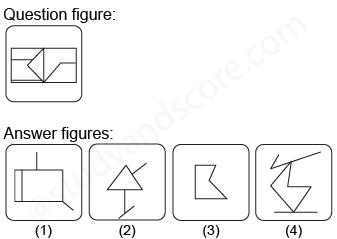Solution: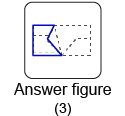Example 2: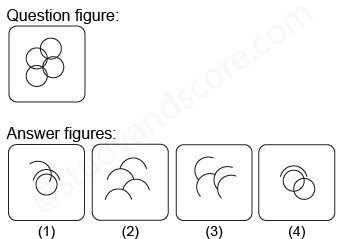Solution: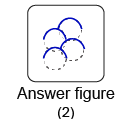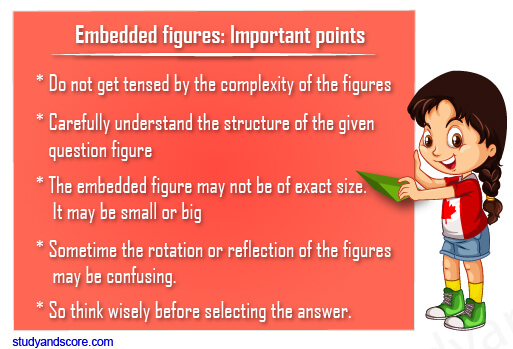Hope you have liked this post.